#Faulted MsFV Example

# Example demonstrating Multiscale Finite Volume Solver over a fault for a corner point grid.

This is an example demonstrating the MsFV solver on a faulted grid. The coarse dual grid should be provided in the file fault_dual.mat and was produced by an inhouse algorithm.

## Load the modules required for the example

mrstModule add coarsegrid msfvm


## Define grid

We define a fine grid of a Cartesian block divided by a sloping fault, with skewed pillars via the GRDECL format. A coarse grid with block size of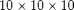is used.

nx = 40; ny = 30; nz = 31;
Nx = 4; Ny = 3; Nz = 3;

% Create grid using deck
grdecl = oneSlopingFault([nx, ny, nz], 5);
G      = processGRDECL(grdecl, 'Verbose', false); clear grdecl;
G      = computeGeometry(G);


## Generate the coarse grid

We process the partition and plot it.

p  = partitionUI(G, [Nx, Ny, Nz]);
p  = processPartition(G, p);
CG = generateCoarseGrid(G, p);
CG = coarsenGeometry(CG);

clf;
plotCellData(G, mod(p, 7), 'edgec', 'k')
view(-10,15); axis tight off;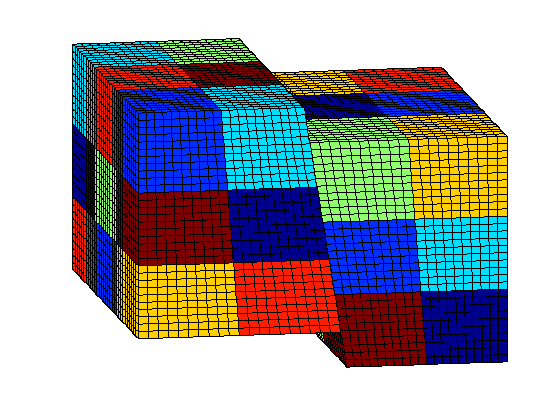## Load the corresponding dual grid

The dual grid was created using an in-house algorithm which will be published at a later date.

load fault_dual


## Visualize the dual grid

We plot the edges between the coarse block centroids

clf;
plotDual(G, DG)
view(0,0); axis tight off;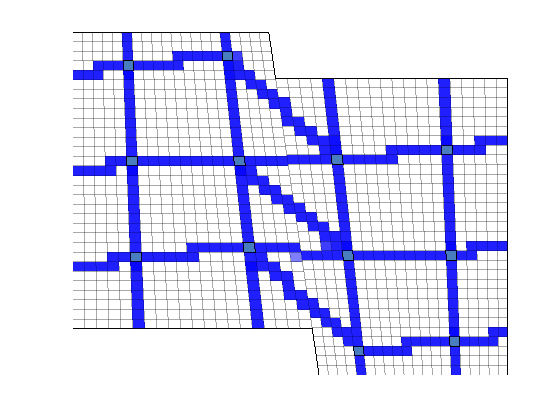## Define permeability and fluid

Disable gravity. Can be enabled if one finds that sort of thing interesting.

gravity off;
% Instansiate a fluid object for one phase flow.
fluid        = initSingleFluid('mu' ,    1*centi*poise     , ...
'rho', 1014*kilogram/meter^3);
% Create layered permeability in logical indices to emulate sedimentary
% rocks across the fault
layers       = [100 400 50 350];
K            = logNormLayers([nx, ny, nz], layers);
rock.perm    = convertFrom(K(1:G.cells.num), milli*darcy);  % mD -> m^2
% Plot the permeability

clf;
plotCellData(G, log10(rock.perm));
view(-10,15); axis tight off;
T = computeTrans(G, rock);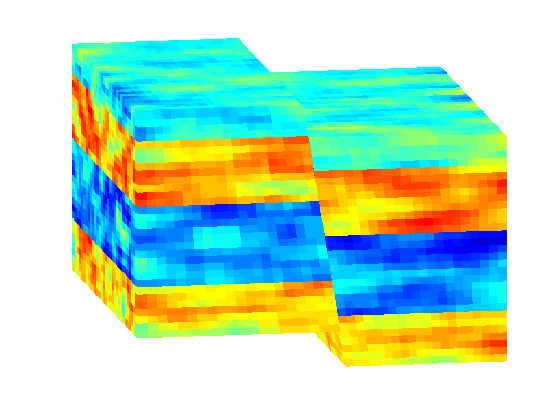## Add a simple Dirichlet pressure boundary

We add boundary conditions along the extremal values of the x axis.

bc = [];
% Find the edges and add unit and zero pressure boundary condition there
d  = abs(G.faces.centroids(:,1) - max(G.faces.centroids(:,1)));
ind1 = find (d < 1e6*eps);
bc = addBC(bc, ind1, 'pressure', 1);

d  = abs(G.faces.centroids(:,1) - min(G.faces.centroids(:,1)));
ind0 = find (d < 1e6*eps);
bc = addBC(bc, ind0, 'pressure', 0);

% Plot the faces selected for boundary conditions, with the largest
% pressure in red and the smallest in red.
clf;
plotGrid(G, 'FaceAlpha', 0, 'EdgeAlpha', .1);
plotFaces(G, ind1, 'FaceColor', 'Red')
plotFaces(G, ind0, 'FaceColor', 'Blue')
view(-10,15); axis tight off;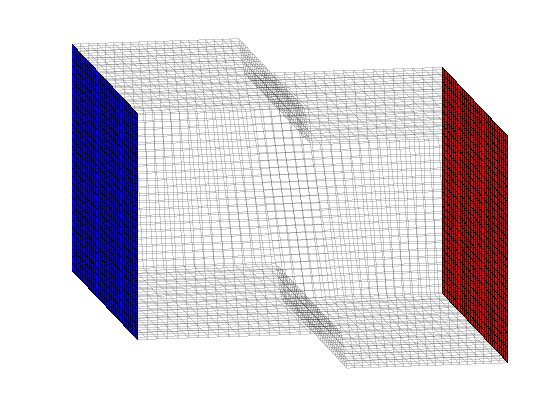## Solve the pressure system

First we initiate a pressure system. This structure is always required, but without transport only the grid is relevant.

sol = initState(G, [], 0, 1);

% Solve TPFA reference solution.
solRef = incompTPFA(sol, G, T, fluid, 'bc', bc);

% Solve multiscale pressure. Reconstruct conservative flow using flow basis
% functions.
solMSFV = solveMSFV_TPFA_Incomp(sol, G, CG, T, fluid, 'Dual', DG, ...
'Reconstruct', true, 'bc', bc, 'Verbose', false, 'SpeedUp', true);


## Plot TPFA solution

clf;
plotCellData(G, solRef.pressure);
view(-10,15); axis tight off; colorbar;
set(gca, 'CLim', [0, max(solRef.pressure)]);
title('TPFA')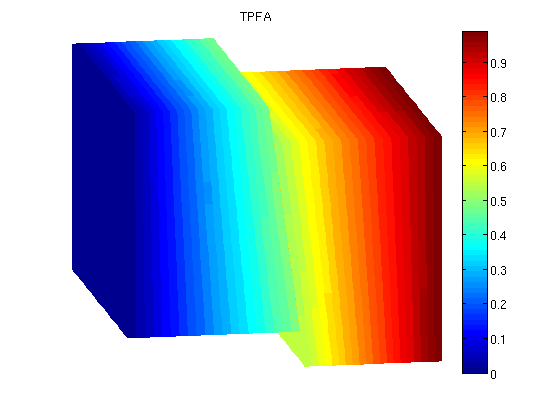## Plot MSFVM solution

clf;
plotCellData(G, solMSFV.pressure);
view(-10,15); axis tight off; colorbar;
title('MsFVM')
% Use same axis scaling as the TPFA solution
set(gca, 'CLim', [0, max(solRef.pressure)]);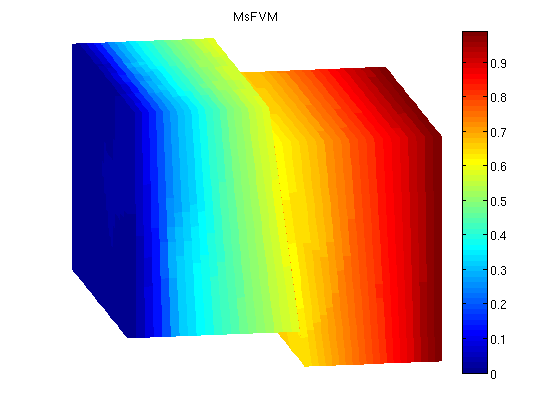## Plot error

reportError(solRef.pressure, solMSFV.pressure);
clf;
% Plot the error scaled with local variation
plotCellData(G, abs(solRef.pressure - solMSFV.pressure) ./ abs(max(solRef.pressure - min(solRef.pressure))));
view(-10,15); axis tight off; colorbar;

ERROR:
2: 0.03061274
Sup: 0.05971864
Minimum 0.00000451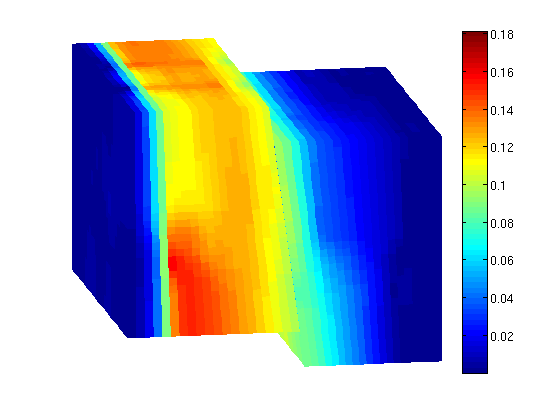Published October 2, 2012